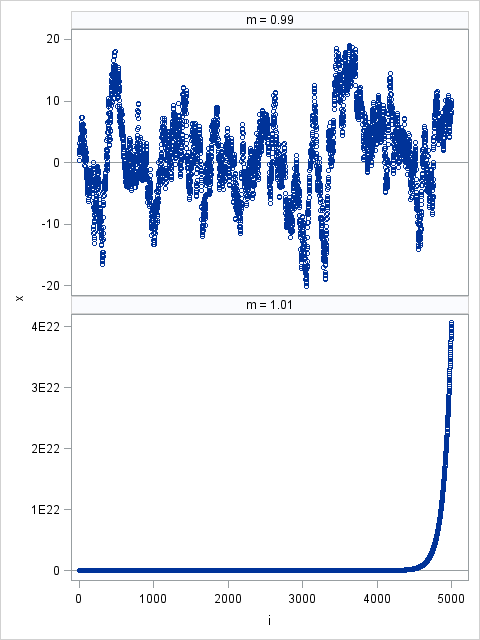A collegue who works with time series sent me the following code snippet. He said that the calculation was overflowing and wanted to know if this was a bug in SAS:

```data A(drop=m); call streaminit(12345); m = 2; x = 0; do i = 1 to 5000; x = m*x + rand("Normal"); output; end; run;```
```NOTE: A number has become too large at line 6 column 11.
The number is >1.80E+308 or <-1.80E+308.
```

This behavior is expected, and is definitely not a bug. The behavior follows from a basic analysis of the computation within the DO loop. Take a moment to figure out why the magnitude of x becomes large in this program.

### A simple discrete dynamical system

Before I became a statistician, I studied dynamical systems, which is the study of the long-term qualitative behavior of a differential equation or of an iterative map. A lot can be learned about the behavior of iterates of x by just ignoring the random term. (You can justify ignoring the random term by noting that rand("Normal") has zero mean.)

The iterative mapping x → mx is linear, so it has simple dynamical properties:

• If m < 1, all initial values for x approach 0 under iteration because the sequence x, mx, m2x, ..., mnx approaches zero for all values of x.
• If m > 1, all nonzero values of x diverge under iteration.

### A discrete dynamical system with a stochastic component

Adding a random term with mean zero does not change the mathematics of this problem very much. However, now the dynamics are described in terms of "expected behavior," because the system is no longer deterministic. For the iterative mapping x → mx + ε, ε ∼ N(0,1), you can expect the following long-term behavior for the iterates:

• the iterates to bounce around a neighborhood of zero when m < 1
• the iterates diverge for m > 1

The following graphs show typical behavior:

```data A; call streaminit(12345); phi = 0.99; x = 0; do i = 1 to 5000; x = phi*x + rand("Normal"); output; end; phi = 1.01; x = 0; do i = 1 to 5000; x = phi*x + rand("Normal"); output; end; run;   proc sgpanel data=A; panelby phi / uniscale=column rows=2; refline 0 / axis=y; scatter x=i y=x; run;```You might wonder about the case m = 1. There is a theorem that says that a random walk x → x + ε, ε ∼ N(0,1), will eventually get arbitrarily far from it's initial position. Therefore the case m = 1 would eventually diverge in finite precision arithmetic...but you might have to wait a REALLY long time!